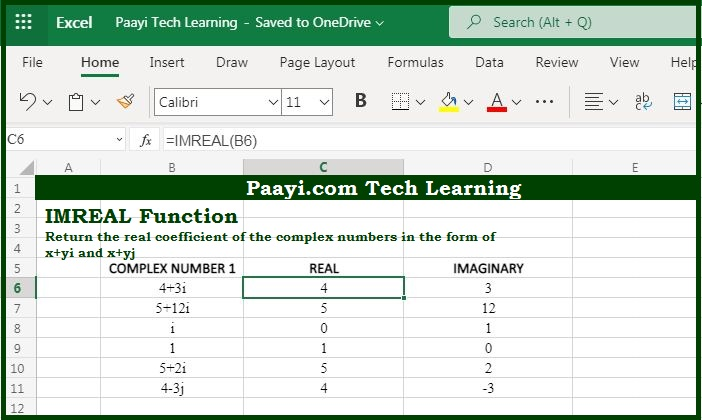# Learn How to Use Microsoft Excel IMREAL Function

Written by | 0 Comments | 613 Views

In this article, you will learn how to use the Microsoft Excel IMREAL function and its prime function in Microsoft Excel. You will also get to know the Microsoft Excel IMREAl function return value and syntax with the help of some examples.

Microsoft Excel IMREAL Function

The prime function of the Microsoft Excel IMREAL function is to get the real coefficient of a complex number. So, with the help of IMREAL function, you can able to return the coefficient of the complex number. The complex number should be in the form of  "x + yi" or "x + yj".

Return Value of IMREAL Function

The return value will be the real coefficient of the complex number.

Syntax of IMREAL Function

=IMREAL(inumber)

Where the arguments:

• inumber: It is a complex number.

## How to Use Microsoft Excel IMREAL Function?As we know that IMREAL function you can able to return the product of one or more complex numbers. The complex number is input in the form text as,  "x + yi" or "x + yj".

It should be noted that only lowercase "i" and "j" is accepted by the IMREAL function and other values will result in a #NUM! error.